# 深入解析 ObjC 中方法的结构

Blog: Draveness

## 方法在内存中的位置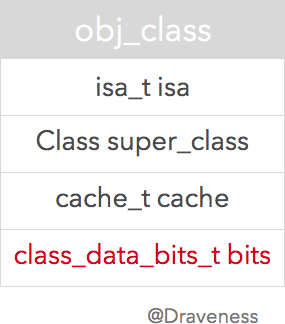• isa 是指向元类的指针，不了解元类的可以看 Classes and Metaclasses
• super_class 指向当前类的父类
• cache 用于缓存指针和 vtable，加速方法的调用
• bits 就是存储类的方法、属性、遵循的协议等信息的地方

### class_data_bits_t 结构体objc_class 结构体中的注释写到 class_data_bits_t 相当于 class_rw_t 指针加上 rr/alloc 的标志。

class_data_bits_t bits;    // class_rw_t * plus custom rr/alloc flags


class_rw_t* data() {
}


bitsFAST_DATA_MASK 进行位运算，只取其中的 [3, 47] 位转换成 class_rw_t * 返回。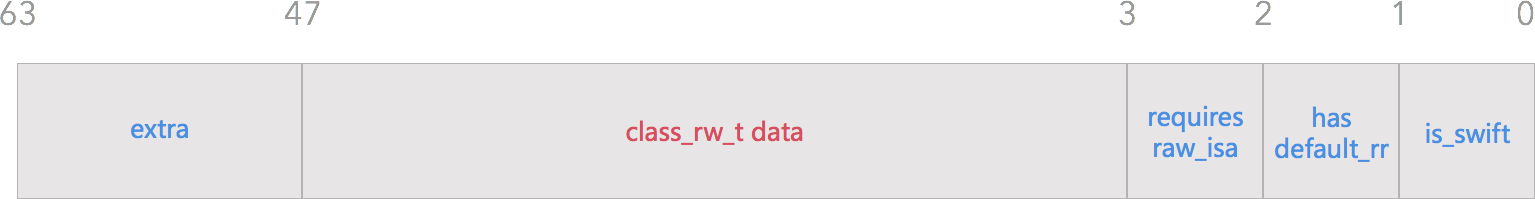#define FAST_IS_SWIFT           (1UL<<0)
#define FAST_HAS_DEFAULT_RR     (1UL<<1)
#define FAST_REQUIRES_RAW_ISA   (1UL<<2)

• isSwift()
• FAST_IS_SWIFT 用于判断 Swift 类
• hasDefaultRR()
• FAST_HAS_DEFAULT_RR 当前类或者父类含有默认的 retain/release/autorelease/retainCount/_tryRetain/_isDeallocating/retainWeakReference/allowsWeakReference 方法
• requiresRawIsa()
• FAST_REQUIRES_RAW_ISA 当前类的实例需要 raw isa

// objc_class 中的 data() 方法
class_data_bits_t bits;

class_rw_t *data() {
return bits.data();
}

// class_data_bits_t 中的 data() 方法
uintptr_t bits;

class_rw_t* data() {
}


### class_rw_t 和 class_ro_t

ObjC 类中的属性、方法还有遵循的协议等信息都保存在 class_rw_t 中：

struct class_rw_t {
uint32_t flags;
uint32_t version;

const class_ro_t *ro;

method_array_t methods;
property_array_t properties;
protocol_array_t protocols;

Class firstSubclass;
Class nextSiblingClass;
};


struct class_ro_t {
uint32_t flags;
uint32_t instanceStart;
uint32_t instanceSize;
uint32_t reserved;

const uint8_t * ivarLayout;

const char * name;
method_list_t * baseMethodList;
protocol_list_t * baseProtocols;
const ivar_list_t * ivars;

const uint8_t * weakIvarLayout;
property_list_t *baseProperties;
};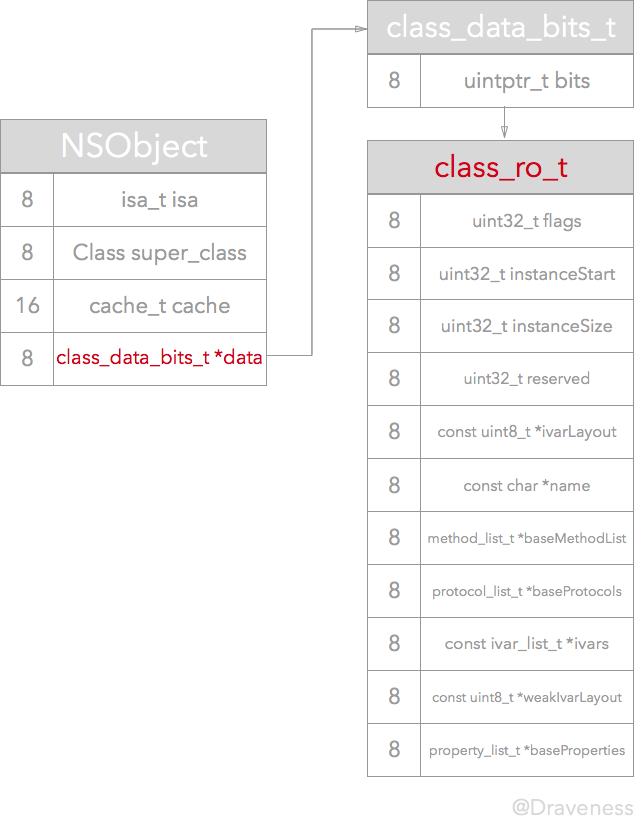1. class_data_bits_t 调用 data 方法，将结果从 class_rw_t 强制转换为 class_ro_t 指针
2. 初始化一个 class_rw_t 结构体
3. 设置结构体 ro 的值以及 flag
4. 最后设置正确的 data
const class_ro_t *ro = (const class_ro_t *)cls->data();
class_rw_t *rw = (class_rw_t *)calloc(sizeof(class_rw_t), 1);
rw->ro = ro;
rw->flags = RW_REALIZED|RW_REALIZING;
cls->setData(rw);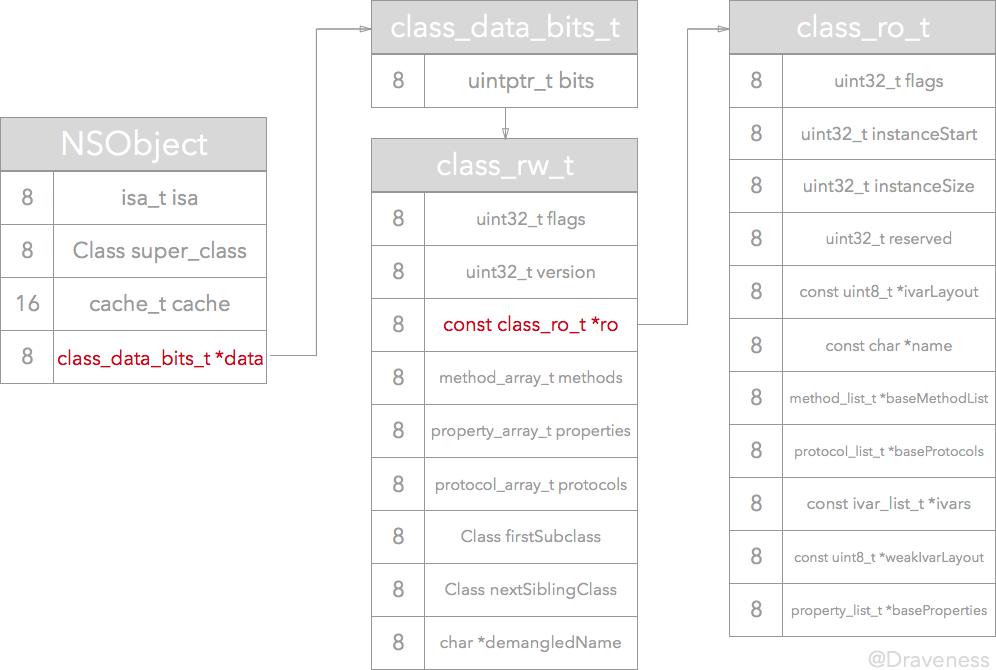## XXObject

// XXObject.h 文件
#import <Foundation/Foundation.h>

@interface XXObject : NSObject

- (void)hello;

@end

// XXObject.m 文件

#import "XXObject.h"

@implementation XXObject

- (void)hello {
NSLog(@"Hello");
}

@end#import <Foundation/Foundation.h>
#import "XXObject.h"

int main(int argc, const char * argv[]) {
@autoreleasepool {
Class cls = [XXObject class];
NSLog(@"%p", cls);
}
return 0;
}


### 编译后内存中类的结构

0x100001168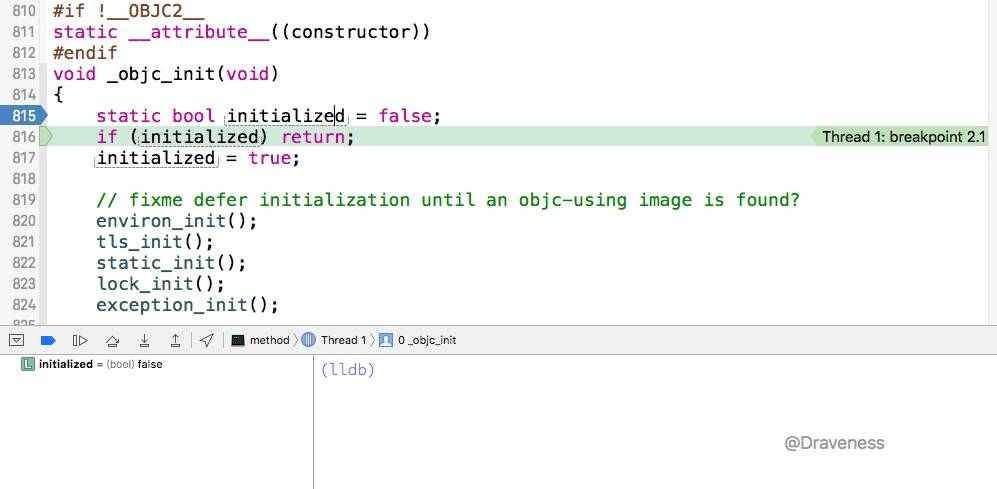(lldb) p (objc_class *)0x100001168
(objc_class *) $0 = 0x0000000100001168 (lldb) p (class_data_bits_t *)0x100001188 (class_data_bits_t *)$1 = 0x0000000100001188
(lldb) p $1->data() warning: could not load any Objective-C class information. This will significantly reduce the quality of type information available. (class_rw_t *)$2 = 0x00000001000010e8
(lldb) p (class_ro_t *)$2 // 将 class_rw_t 强制转化为 class_ro_t (class_ro_t *)$3 = 0x00000001000010e8
(lldb) p *$3 (class_ro_t)$4 = {
flags = 128
instanceStart = 8
instanceSize = 8
reserved = 0
ivarLayout = 0x0000000000000000 <no value available>
name = 0x0000000100000f7a "XXObject"
baseMethodList = 0x00000001000010c8
baseProtocols = 0x0000000000000000
ivars = 0x0000000000000000
weakIvarLayout = 0x0000000000000000 <no value available>
baseProperties = 0x0000000000000000
}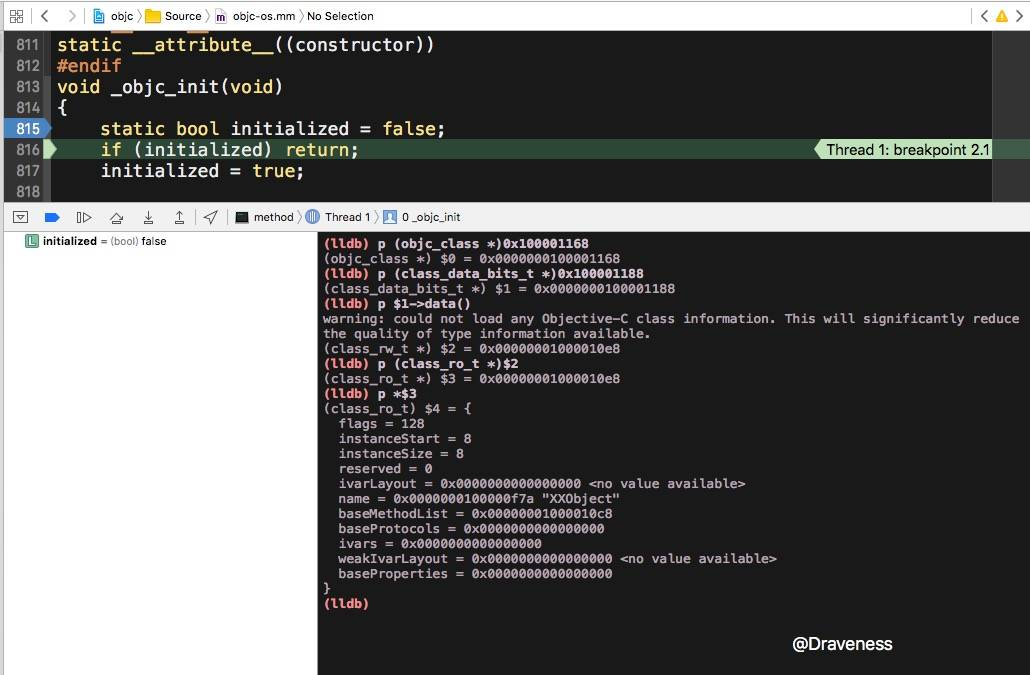(class_ro_t) $4 = { flags = 128 instanceStart = 8 instanceSize = 8 reserved = 0 ivarLayout = 0x0000000000000000 <no value available> name = 0x0000000100000f7a "XXObject" baseMethodList = 0x00000001000010c8 baseProtocols = 0x0000000000000000 ivars = 0x0000000000000000 weakIvarLayout = 0x0000000000000000 <no value available> baseProperties = 0x0000000000000000 }  可以看到这里面只有 baseMethodListname 是有值的，其它的 ivarLayoutbaseProtocolsivarsweakIvarLayoutbaseProperties 都指向了空指针，因为类中没有实例变量，协议以及属性。所以这里的结构体符合我们的预期。 通过下面的命令查看 baseMethodList 中的内容： (lldb) p$4.baseMethodList
(method_list_t *) $5 = 0x00000001000010c8 (lldb) p$5->get(0)
(method_t) $6 = { name = "hello" types = 0x0000000100000fa4 "v16@0:8" imp = 0x0000000100000e90 (method-[XXObject hello] at XXObject.m:13) } (lldb) p$5->get(1)
Assertion failed: (i < count), function get, file /Users/apple/Desktop/objc-runtime/runtime/objc-runtime-new.h, line 110.
error: Execution was interrupted, reason: signal SIGABRT.
The process has been returned to the state before expression evaluation.
(lldb)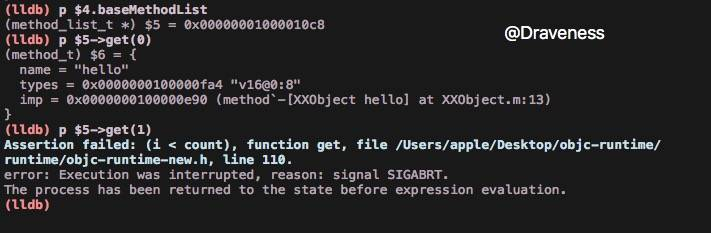(lldb) p (class_data_bits_t *)0x0000000100001188 // 在类指针上加 32 的 offset 打印 class_data_bits_t 指针
(class_data_bits_t *) $263 = 0x0000000100001188 (lldb) p *$263 // 访问 class_data_bits_t 指针的内容
(class_data_bits_t) $264 = (bits = 4302315312) (lldb) p$264.data() // 获取 class_rw_t
(class_rw_t *) $265 = 0x0000000100701f30 (lldb) p *$265 // 访问 class_rw_t 指针的内容，发现它的 ro 已经设置好了
(class_rw_t) $266 = { flags = 2148007936 version = 0 ro = 0x00000001000010e8 methods = { list_array_tt<method_t, method_list_t> = { = { list = 0x0000000000000000 arrayAndFlag = 0 } } } properties = { list_array_tt<property_t, property_list_t> = { = { list = 0x0000000000000000 arrayAndFlag = 0 } } } protocols = { list_array_tt<unsigned long, protocol_list_t> = { = { list = 0x0000000000000000 arrayAndFlag = 0 } } } firstSubclass = nil nextSiblingClass = nil demangledName = 0x0000000000000000 <no value available> } (lldb) p$266.ro // 获取 class_ro_t 指针
(const class_ro_t *) $267 = 0x00000001000010e8 (lldb) p *$267 // 访问 class_ro_t 指针的内容
(const class_ro_t) $268 = { flags = 128 instanceStart = 8 instanceSize = 8 reserved = 0 ivarLayout = 0x0000000000000000 <no value available> name = 0x0000000100000f7a "XXObject" baseMethodList = 0x00000001000010c8 baseProtocols = 0x0000000000000000 ivars = 0x0000000000000000 weakIvarLayout = 0x0000000000000000 <no value available> baseProperties = 0x0000000000000000 } (lldb) p$268.baseMethodList // 获取基本方法列表
(method_list_t *const) $269 = 0x00000001000010c8 (lldb) p$269->get(0) // 访问第一个方法
(method_t) $270 = { name = "hello" types = 0x0000000100000fa4 "v16@0:8" imp = 0x0000000100000e90 (method-[XXObject hello] at XXObject.m:13) } (lldb) p$269->get(1) // 尝试访问第二个方法，越界
error: Execution was interrupted, reason: signal SIGABRT.
The process has been returned to the state before expression evaluation.
Assertion failed: (i < count), function get, file /Users/apple/Desktop/objc-runtime/runtime/objc-runtime-new.h, line 110.
(lldb)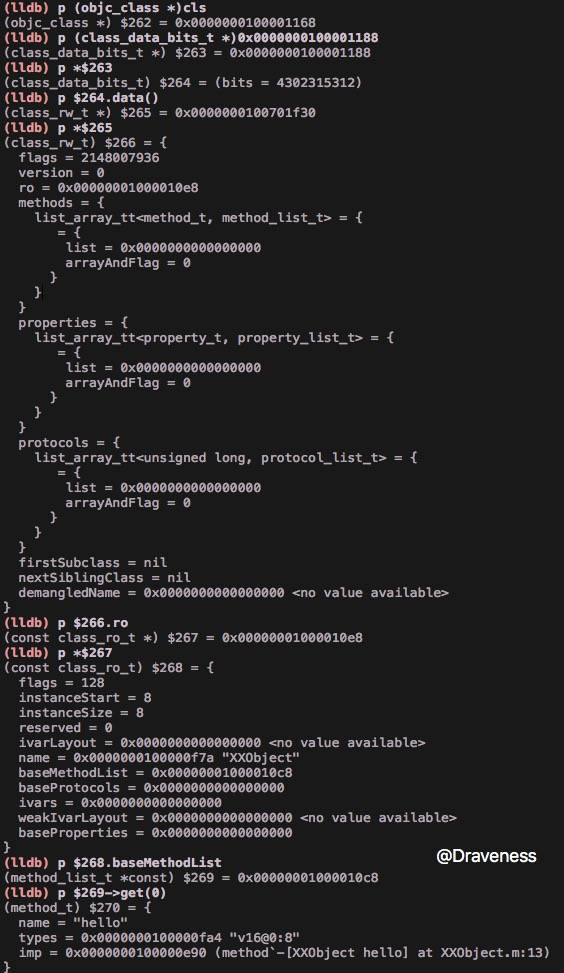const class_ro_t *ro = (const class_ro_t *)cls->data();
class_rw_t *rw = (class_rw_t *)calloc(sizeof(class_rw_t), 1);
rw->ro = ro;
rw->flags = RW_REALIZED|RW_REALIZING;
cls->setData(rw);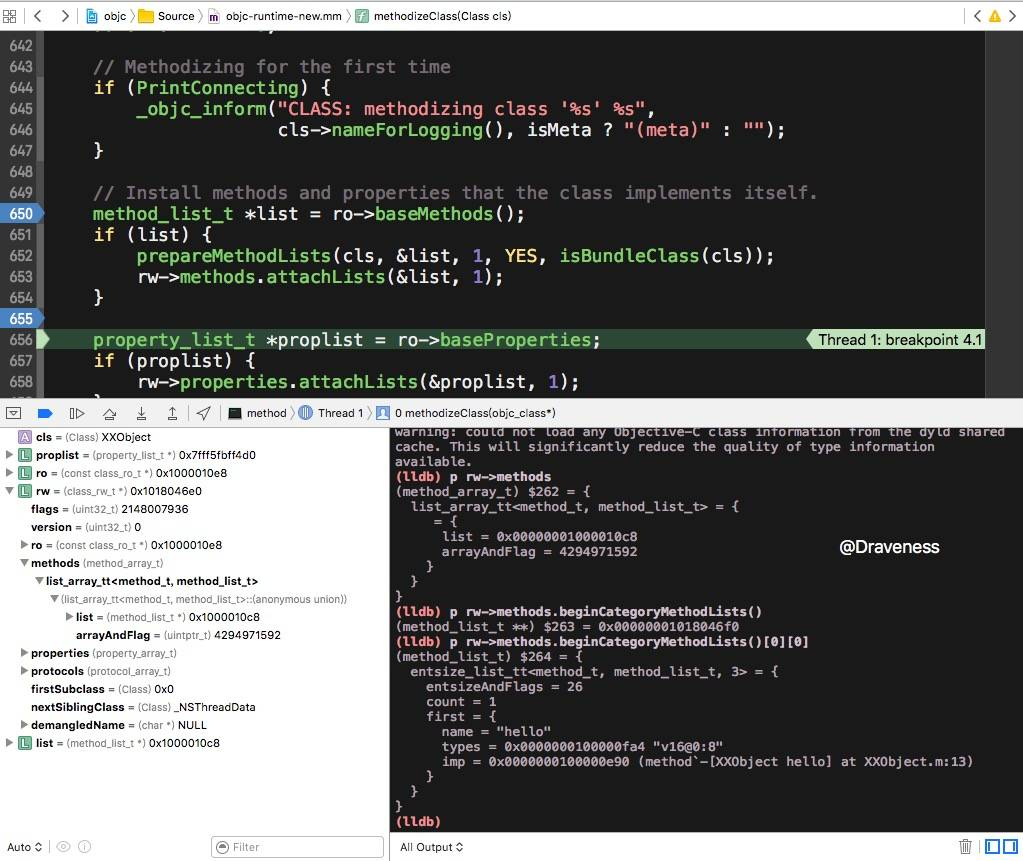## 方法的结构

struct method_t {
SEL name;
const char *types;
IMP imp;
};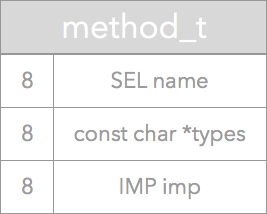name = "hello"
types = 0x0000000100000fa4 "v16@0:8"
imp = 0x0000000100000e90 (method-[XXObject hello] at XXObject.m:13


## 小结

1. 类在内存中的位置是在编译期间决定的，在之后修改代码，也不会改变内存中的位置。
2. 类的方法、属性以及协议在编译期间存放到了“错误”的位置，直到 realizeClass 执行之后，才放到了 class_rw_t 指向的只读区域 class_ro_t，这样我们即可以在运行时为 class_rw_t 添加方法，也不会影响类的只读结构。
3. class_ro_t 中的属性在运行期间就不能改变了，再添加方法时，会修改 class_rw_t 中的 methods 列表，而不是 class_ro_t 中的 baseMethods`，对于方法的添加会在之后的文章中分析。

## 参考资料### 转载申请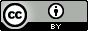### Go 语言设计与实现